# survParamSim

library(dplyr)
#>
#> Attaching package: 'dplyr'
#> The following objects are masked from 'package:stats':
#>
#>     filter, lag
#> The following objects are masked from 'package:base':
#>
#>     intersect, setdiff, setequal, union
library(ggplot2)
library(survival)
library(survParamSim)

set.seed(12345)

## Fit model with survreg() function

Before running a parametric survival simulation, you need to fit a model to your data using survreg() function of survival package.
In this vignette, we will be using colon dataset available in survival package, where the treatment effect of adjuvant Levamisole+5-FU for colon cancer over placebo is evaluated.

First, we load the data and do some data wrangling.

# ref for dataset https://vincentarelbundock.github.io/Rdatasets/doc/survival/colon.html
colon2 <-
as_tibble(colon) %>%
# recurrence only and not including Lev alone arm
filter(rx != "Lev",
etype == 1) %>%
# Same definition as Lin et al, 1994
mutate(rx = factor(rx, levels = c("Obs", "Lev+5FU")),
depth = as.numeric(extent <= 2))

Generating Kaplan-Meier curves for visually checking the data.
The second plot is looking at how many censoring we have over time.
Looks like we have a fairly uniform censoring between 1800 to 3000 days.

survfit.colon <- survfit(Surv(time, status) ~ rx, data = colon2)
survminer::ggsurvplot(survfit.colon)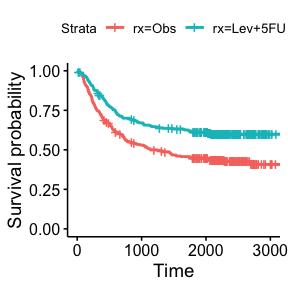survfit.colon.censor <- survfit(Surv(time, 1-status) ~ rx, data = colon2)
survminer::ggsurvplot(survfit.colon.censor)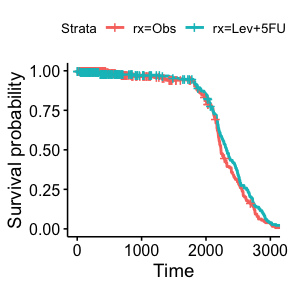Next we fit a lognormal parametric model for the data.
Here we are using node4 and depth as additional covariates in addition to treatment (rx).
You can see that all of the factor has strong association with the outcome.

fit.colon <- survreg(Surv(time, status) ~ rx + node4 + depth,
data = colon2, dist = "lognormal")

summary(fit.colon)
#>
#> Call:
#> survreg(formula = Surv(time, status) ~ rx + node4 + depth, data = colon2,
#>     dist = "lognormal")
#>               Value Std. Error     z       p
#> (Intercept)  7.5103     0.1343 55.92 < 2e-16
#> rxLev+5FU    0.7606     0.1677  4.54 5.7e-06
#> node4       -1.3474     0.1816 -7.42 1.2e-13
#> depth        1.1243     0.2661  4.22 2.4e-05
#> Log(scale)   0.6040     0.0461 13.10 < 2e-16
#>
#> Scale= 1.83
#>
#> Log Normal distribution
#> Loglik(model)= -2561.7   Loglik(intercept only)= -2607.6
#>  Chisq= 91.8 on 3 degrees of freedom, p= 9e-20
#> Number of Newton-Raphson Iterations: 4
#> n= 619

## Perform simulation

surv_param_sim() is the main function of the package that takes survreg object as described above.
It also require you to supply newdata, which is required even if it is not new - i.e. the same data was used for both survreg() and surv_param_sim().

What it does is: 1. Re-sample all the coefficients in the parametric survival model from variance-covariance matrix for n.rep times. 2. Perform survival time for all subjects in newdata with the corresponding covariates, using one of the resampled coefficients. Also generate censoring time according to censor.dur (if not NULL), and replace the simulated survival time above if censoring time is earlier. 4. Repeat the steps 2. for n.rep times.

sim <-
surv_param_sim(object = fit.colon, newdata = colon2,
# Simulate censoring according to the plot above
censor.dur = c(1800, 3000),
# Simulate only 100 times to make the example go fast
n.rep = 100)

After the simulation is performed, you can either extract raw simulation results or further calculate Kaplan-Meier estimates or hazard ratio of treatment effect, as you see when you type sim in the console.

sim
#> ---- Simulated survival data with the following model ----
#> survreg(formula = Surv(time, status) ~ rx + node4 + depth, data = colon2,
#>     dist = "lognormal")
#>
#> * Use extract_sim() function to extract individual simulated survivals
#> * Use calc_km_pi() function to get survival curves and median survival time
#> * Use calc_hr_pi() function to get hazard ratio
#>
#> * Settings:
#>     #simulations: 100
#>     #subjects: 619 (without NA in model variables)

## Survival time profile

To calculate survival curves for each simulated dataset, calc_km_pi() can be used on the simulated object above.

km.pi <- calc_km_pi(sim, trt = "rx")
km.pi
#> ---- Simulated and observed (if calculated) survival curves ----
#> * Use extract_median_surv() to extract median survival times
#> * Use extract_km_pi() to extract prediction intervals of K-M curves
#> * Use plot_km_pi() to draw survival curves
#>
#> * Settings:
#>     trt: rx
#>     group: (NULL)
#>     pi.range: 0.95
#>     calc.obs: TRUE

Similar to the raw simulated object, you can have a few options for further processing - one of them is plotting prediction intervals with plot_km_pi() function.

km.pi
#> ---- Simulated and observed (if calculated) survival curves ----
#> * Use extract_median_surv() to extract median survival times
#> * Use extract_km_pi() to extract prediction intervals of K-M curves
#> * Use plot_km_pi() to draw survival curves
#>
#> * Settings:
#>     trt: rx
#>     group: (NULL)
#>     pi.range: 0.95
#>     calc.obs: TRUE
plot_km_pi(km.pi) +
theme(legend.position = "bottom") +
labs(y = "Recurrence free rate") +
expand_limits(y = 0)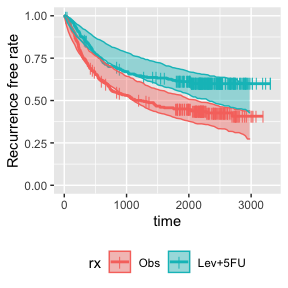Or providing median survival summary table with extract_median_surv() function.

extract_median_surv(km.pi)
#> # A tibble: 8 x 5
#>   rx          n description median quantile
#>   <fct>   <dbl> <chr>        <dbl>    <dbl>
#> 1 Obs       315 pi_low       1108.    0.025
#> 2 Obs       315 pi_med       1538.    0.5
#> 3 Obs       315 pi_high      2113.    0.975
#> 4 Obs       315 obs          1236    NA
#> 5 Lev+5FU   304 pi_low       2126.    0.025
#> 6 Lev+5FU   304 pi_med       2697.    0.5
#> 7 Lev+5FU   304 pi_high      2927.    0.975
#> 8 Lev+5FU   304 obs            NA    NA

Plot can also be made for subgroups.
You can see that prediction interval is wide for (depth: 1 & nodes4: 1) group, mainly due to small number of subjects

km.pi <- calc_km_pi(sim, trt = "rx", group = c("node4", "depth"))

plot_km_pi(km.pi) +
theme(legend.position = "bottom") +
labs(y = "Recurrence free rate") +
expand_limits(y = 0)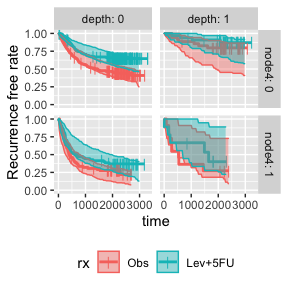## Hazard ratios (HRs)

To calculate prediction intervals of HRs, calc_hr_pi() can be used on the simulated object above. Here I only generated subgroups based on “depth”, since the very small N in (depth: 1 & nodes4: 1) can cause issue with calculating HRs.

hr.pi <- calc_hr_pi(sim, trt = "rx", group = c("depth"))

hr.pi
#> ---- Simulated and observed (if calculated) hazard ratio ----
#> * Use extract_hr_pi() to extract prediction intervals and observed HR
#> * Use extract_hr() to extract individual simulated HRs
#> * Use plot_hr_pi() to draw histogram of predicted HR
#>
#> * Settings:
#>     trt: rx
#>          (Lev+5FU as test trt, Obs as control)
#>     group: depth
#>     pi.range: 0.95
#>     calc.obs: TRUE
plot_hr_pi(hr.pi)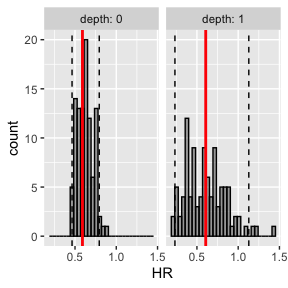You can also extract prediction intervals and observed HR with extract_hr_pi() function.

extract_hr_pi(hr.pi)
#> # A tibble: 8 x 4
#>   depth description    HR quantile
#>   <dbl> <chr>       <dbl>    <dbl>
#> 1     0 pi_low      0.464    0.025
#> 2     0 pi_med      0.624    0.5
#> 3     0 pi_high     0.794    0.975
#> 4     0 obs         0.590   NA
#> 5     1 pi_low      0.233    0.025
#> 6     1 pi_med      0.597    0.5
#> 7     1 pi_high     1.13     0.975
#> 8     1 obs         0.607   NA